# 2 dimensions of portfolio diversity

Portfolio diversity is a balancing act.

## Previously

The post “Portfolio diversity” talked about the role of the correlation between assets and the portfolio.  The current post fills a hole in that post.

## The 2 dimensions

#### asset-portfolio correlation

Each asset in the universe has a correlation with the portfolio.  If there are any assets that have a very high correlation with the portfolio, then the portfolio can not be considered to be well-diversified — it acts like that single asset.

#### risk fraction

If the portfolio returns are strongly driven by a single asset, then the portfolio is not well-diversified.  A reasonable measure of that is the fraction of the portfolio variance that each asset accounts for.

Often the weight of the assets has been used.  That’s not terrible, but it is an indirect measure of what we really want.

## Examples

We use the same set-up as the previous post.

### Data

We use (almost all of) the S&P 500 constituents as of the start of 2011.

The variance matrix of the assets is a Ledoit-Wolf shrinkage estimate based on daily data during 2010.

### Constraints

We generate a few sets of random portfolios given some constraints.  We generate portfolios containing 20 stocks and others containing 200 stocks.

The base constraints for the 20-stock portfolios are:

• long-only
• exactly 20 names
• maximum weight is 10%
• minimum weight is 1%

The base constraints for the 200-name portfolios are:

• long-only
• exactly 200 names
• maximum weight is 1%
• minimum weight is 0.1%

### Pictures

Figures 1 and 2 show the (ex-ante) maximum asset-portfolio correlation versus the maximum variance fraction for random portfolios that were generated with the constraints described above.

Figure 1: Maximum asset-portfolio correlation versus maximum variance fraction for 20-name random portfolios.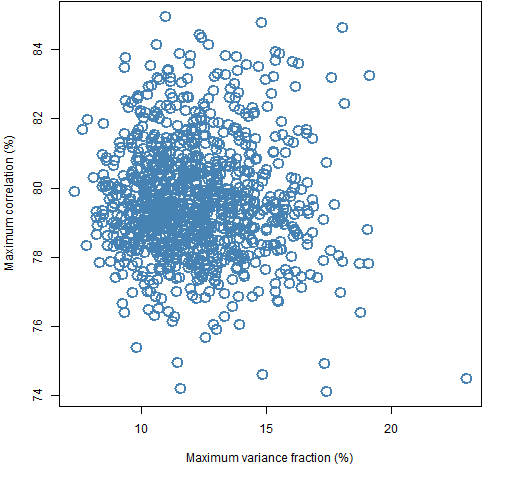The maximum weight is limited to 10% but there is one portfolio that has a variance fraction that is well over 20%.

Figure 2: Maximum asset-portfolio correlation versus maximum variance fraction for 200-name random portfolios.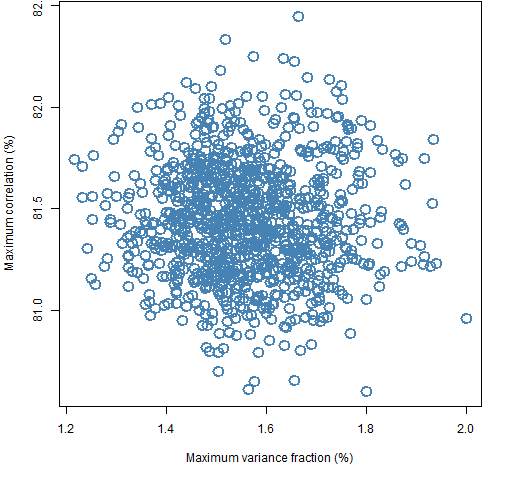We usually think of increasing the number of assets in our portfolio as diversifying.  In terms of variance fraction, we see that happening.  However, in terms of correlation the effect is slightly towards anti-diversification.

We can try to force such diversification by adding a constraint to make the maximum asset-portfolio correlation no more than 75%.  That is easily achieved in the 20-stock portfolios, and the effect on variance fraction is minimal as can be seen in Figure 3.

Figure 3: Distribution of maximum variance fraction for 20-name portfolios: base constraints (blue), base constraints plus maximum correlation no more than 75% (gold).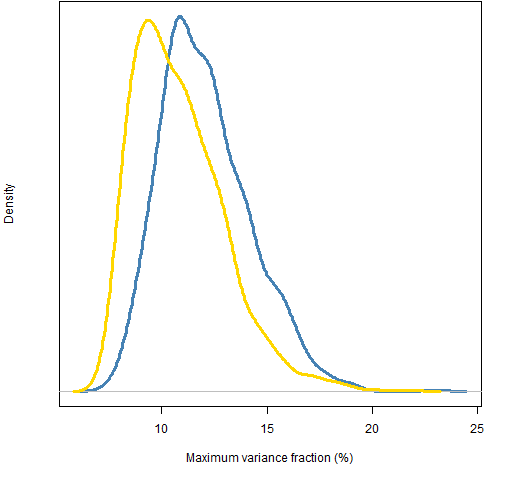Intriguingly adding the correlation constraint slightly reduces the maximum variance fraction.

In contrast, achieving the correlation constraint in the 200-stock portfolios was more challenging, and has a definite effect on the variance fraction (Figure 4).

Figure 4: Distribution of maximum variance fraction for 200-name portfolios: base constraints (blue), base constraints plus maximum correlation no more than 75% (gold).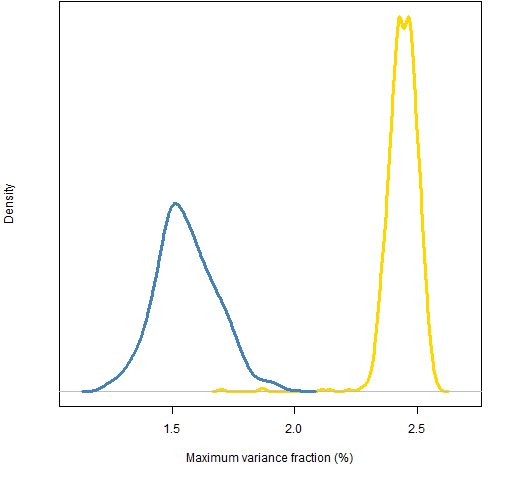## Dimensional connection

How connected are asset-portfolio correlation and variance fraction?

Figures 5 and 6 look at the correlation and variance fraction for each asset in the universe for the random portfolio that happened to be first in each set using only the base constraints.

Figure 5: Asset-portfolio correlation versus asset variance fraction for the first 20-name random portfolio.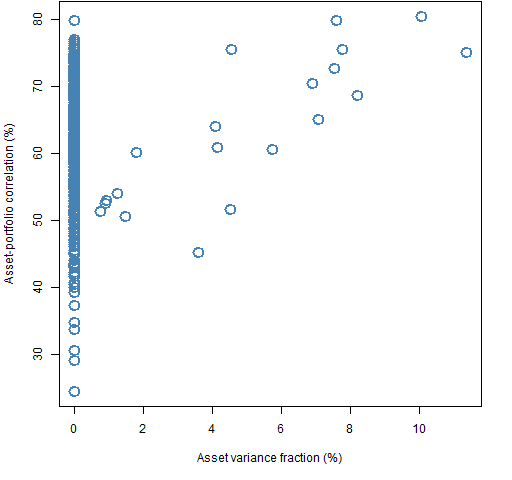Figure 6: Asset-portfolio correlation versus asset variance fraction for the first 200-name random portfolio.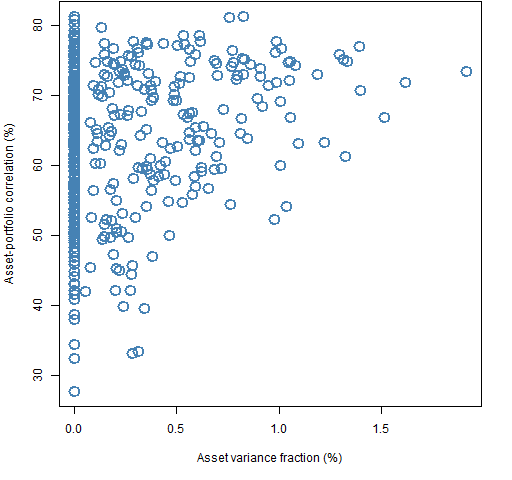The general pattern is a triangle shape.  The correlation varies widely for assets not in the portfolio (including the possibility of being the largest correlation).  But the minimum possible correlation seems to increase as the variance fraction increases.

Portfolio diversity must be the topic of the day as the post “Diversification and Risk Reduction” just appeared on CSS Analytics.

## Summary

• Portfolio diversity is more interesting than it first appears
• I don’t think diversity has a single dimension

## Appendix R

The computations were done in R.

#### generate random portfolios

The random portfolios are created with the Portfolio Probe software.  The command uses a feature that is new in version 1.05, though this analysis could be done (less conveniently) in version 1.04 as well.

The 200-name portfolios were generated with:

```require(PortfolioProbe)

div2rp.200w <- random.portfolio(1000, sp5.price10,
sp5.var10, gross=1e7, long.only=TRUE,
max.weight=.01, threshold=1e7 * .001/sp5.price10,
port.size=c(200,200), risk.fraction=list(1,1),
rf.style=c("fraction", "corport"))```

The “1000” is specifying the number of portfolios to be generated. This command “imposes” constraints on the maximum variance fraction and maximum correlation of 1.  These are not constraining at all, but they make the next step slightly easier.

The result is a list where each component represents one of the portfolios and gives the number of shares held for each name in that portfolio.

#### retrieve asset statistics

The following command gives us a list where each component contains — for each asset in the universe — the variance fraction and correlation with the portfolio.

```div2rp.200w.riskfrac <- randport.eval(div2rp.200w,
keep="risk.fraction")```

#### collect maximums

At the moment we just care about the maximum within each portfolio of each of the two quantities.  We throw away everything but the maximums and put them into a matrix that is 1000 rows (the number of random portfolios) by 2 columns.

```div2rp.200w.max <- do.call('rbind', lapply(
div2rp.200w.riskfrac, function(x)
apply(x\$risk.fraction, 2, max)))```

#### constraint difficulty

We can measure how hard adding the 75% correlation constraint was in the 200-name case:

```> attr(div2rp.200w75c, "funevals") / attr(div2rp.200w, "funevals")
 205.0481```

So the algorithm had to work 200 times harder to find suitable portfolios.  The equivalent value for the 20-name portfolios is about 21.

What we’d really like to know is what fraction of the portfolios that obey the base constraints also obey the added constraint.  That seems like a challenging thing to learn unless the probability is fairly large.  In the case of this particular constraint for 200 names, the probability appears to be much, much less than one in a thousand.

This entry was posted in Quant finance, R language and tagged , , , , . Bookmark the permalink.